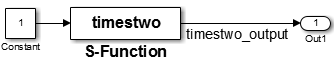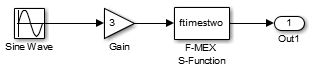## Inline S-Functions

Writing S-functions to be included in generated code involves requirements that go beyond writing S-functions used only for simulation. Before you proceed to inline an S-function make sure that it meets requirements and functions as you expect. For more information, see S-Functions and Code Generation. If your S-function is multirate, see Time-Based Scheduling and Code Generation and Modeling for Multitasking Execution, and Rate Grouping Compliance and Compatibility Issues (Embedded Coder).

### Inline S-Functions with Block Target Files

#### When to Inline S-Functions

With C MEX S-functions, non-ERT targets support calling the original C MEX code if the source code (`.c` file) is available when entering the build phase. For S-functions that are in Fortran or MATLAB® language, you must inline them to have complete code generation for Simulink® models that contain them. Additionally, once you have determined that you will inline an S-function, you must decide to make it either fully inlined or wrapped.

#### Fully Inlined S-Functions

The block target file for a fully inlined S-function is a self-contained definition of how to inline the block’s functionality directly into the various portions of the generated code — start code, output code, etc. This approach is most beneficial when there are many modes and data types supported for algorithms that are relatively small or when the code size is not significant.

#### Function-Based or Wrapped Code Generation

When the physical size of the code for a block becomes too large for inlining, the block target file is written to gather inputs, outputs, and parameters, and make a call to a function that you write to perform the block functionality. This has an advantage in generated code size when the code in the function is large or there are many instances of this block in a model. Of course, you should consider the overhead of the function call when weighing the option of fully inlining the block algorithm or generating function calls.

If you choose to go with function-based code generation, two more options need consideration:

• Write the functions once, put them in `.c` files, and have the TLC code’s `BlockTypeSetup` method specify external references to your support functions. Use `LibAddToModelSources` for names of the modules containing the supporting functions. This approach is usually done using one function per file to get the smallest executable possible.

• Write a more sophisticated TLC file. In addition to methods such as `Start` and `Outputs`, conditionally generate customized versions of functions (data types, widths, algorithms, and so on), in separate code generation buffers, to be written to a separate `.c` file. The file should contain only functions used by this model, instead of all possible functions.

Either approach can produce optimal code. The first option can result in hundreds of files if your S-function supports many data types, signal widths, and algorithm choices. The second approach is more difficult to write, but results in a more maintainable code generation library, and the code can be every bit as tight as the first approach.

For further information on wrapping, see Wrapper Inlined S-Function Example.

### Inline MATLAB File S-Functions

You can inline the functionality of MATLAB file S-functions in the generated code. The process for writing a block target file for a MATLAB file S-function is essentially identical to the process for writing a C MEX S-function.

Note

While you can fully inline a MATLAB file S-function to improve performance, Simulink Accelerator™ or the code generator does not include a C or C++ API for the MATLAB Math Library. You therefore cannot call MATLAB Math Library functions from a TLC file.

The following example illustrates the equivalence of C MEX and MATLAB file S-functions for code generation. The S-function MATLAB file `timestwo.m` is equivalent to the C MEX S-function `timestwo`. The TLC file for the C MEX S-function `timestwo` works for the S-function MATLAB file `timestwo.m`. TLC is independent of the type of S-function because TLC requires only the root name of the S-function and not its type. In the case of `timestwo`, one line determines how the code generator implements the TLC file:

`%implements "timestwo" "C"`

To try this yourself:

1. Create the following sample model:2. Copy the file `timestwo.m` from the folder `matlabroot/toolbox/simulink/simdemos/simfeatures` (open) to a temporary folder.

3. Copy the file `timestwo.tlc` from the folder `matlabroot/toolbox/simulink/simdemos/simfeatures/tlc_c` (open) to the same temporary folder.

4. In MATLAB, change folder (`cd`) to the temporary folder and make a Simulink model with an S-function block that calls `timestwo.`

5. On the Signal Attributes tab of the Inport Block Parameters dialog box, set the Port dimensions parameter to `5`.

Simulink uses the MATLAB file S-function for simulation because the MATLAB search path finds `timestwo.m` in the current folder before finding the C MEX S-function `timestwo` in the `matlabpath`. Verify which S-function the code generator uses by typing the MATLAB command:

`which timestwo`

The answer is the MATLAB file S-function `timestwo.m` in the temporary folder.

In the generated code, the `timestwo.tlc` file inlines the MATLAB file S-function.

```/* S-Function (timestwo): '<Root>/MATLAB S-Function' */ /* Multiply input by two */ { int_T i1; const real_T *u0 = &times2_B.Gain; real_T *y0 = &times2_Y.Out1; for (i1=0; i1 < 5; i1++) { y0[i1] = u0[i1] * 2.0; } }```

The output is the product of each input, `u0[i1]` times 2.0. The code generator uses this `Outputs` method from the block target file to generate code:

```%function Outputs(block, system) Output /* %<Type> Block: %<Name> */ %% /* Multiply input by two */ %assign rollVars = ["U", "Y"] %roll idx = RollRegions, lcv = RollThreshold, block, "Roller", rollVars %<LibBlockOutputSignal(0, "", lcv, idx)> = \ %<LibBlockInputSignal(0, "", lcv, idx)> * 2.0; %endroll %endfunction```

Alter these temporary copies of the MATLAB file S-function and the TLC file to see how they interact. Start out by just changing the comments in the TLC file and see the changes that appear in the generated code. Then, work up to algorithmic changes.

For more information on inlining C MEX S-Functions, see Inline C MEX S-Functions.

### Inline Fortran (F-MEX) S-Functions

The capabilities of Fortran MEX S-functions can be fully inlined using a TLC block target file. This interface can be illustrated with a Fortran MEX S-function that implements the `timestwo` function. Here is the sample Fortran S-function code:

```C C FTIMESTWO.FOR C C C A sample FORTRAN representation of a C timestwo S-function. C Copyright 1990-2000 The MathWorks, Inc. C C===================================================== C Function: SIZES C C Abstract: C Set the size vector. C C SIZES returns a vector which determines model C characteristics. This vector contains the C sizes of the state vector and other C parameters. More precisely, C SIZE(1) number of continuous states C SIZE(2) number of discrete states C SIZE(3) number of outputs C SIZE(4) number of inputs C SIZE(5) number of discontinuous roots in C the system C SIZE(6) set to 1 if the system has direct C feedthrough of its inputs, C otherwise 0 C C===================================================== SUBROUTINE SIZES(SIZE) C .. Array arguments .. INTEGER*4 SIZE(*) C .. Parameters .. INTEGER*4 NSIZES PARAMETER (NSIZES=6) SIZE(1) = 0 SIZE(2) = 0 SIZE(3) = 1 SIZE(4) = 1 SIZE(5) = 0 SIZE(6) = 1 RETURN END C C===================================================== C Function: OUTPUT C C Abstract: C Perform output calculations for continuous C signals. C===================================================== C .. Parameters .. SUBROUTINE OUTPUT(T, X, U, Y) REAL*8 T REAL*8 X(*), U(*), Y(*) Y(1) = U(1) * 2.0 RETURN END C C===================================================== C Stubs for unused functions. C===================================================== SUBROUTINE INITCOND(X0) REAL*8 X0(*) C --- Nothing to do. RETURN END SUBROUTINE DERIVS(T, X, U, DX) REAL*8 T, X(*), U(*), DX(*) C --- Nothing to do. RETURN END SUBROUTINE DSTATES(T, X, U, XNEW) REAL*8 T, X(*), U(*), XNEW(*) C --- Nothing to do. RETURN END SUBROUTINE DOUTPUT(T, X, U, Y) REAL*8 T, X(*), U(*), Y(*) C --- Nothing to do. RETURN END SUBROUTINE TSAMPL(T, X, U, TS, OFFSET) REAL*8 T,TS,OFFSET,X(*),U(*) C --- Nothing to do. RETURN END SUBROUTINE SINGUL(T, X, U, SING) REAL*8 T, X(*), U(*), SING(*) C --- Nothing to do. RETURN END```

Copy the preceding code into file `ftimestwo.for` in a convenient working folder.

Putting this into an S-function block in a simple model will illustrate the interface for inlining the S-function. Once your Fortran MEX environment is set up, prepare the code for use by compiling the S-function in a working folder along with the file `simulink.for` from the folder `matlabroot/simulink/src` (open). For more information about setting up your Fortran MEX environment, see Create Level-2 Fortran S-Functions.

Compile the code with the `mex` command at the MATLAB command line:

`mex ftimestwo.for simulink.for`

Now reference this block from a simple model set with a fixed-step solver and the `grt` target.The TLC code for inlining this block is a modified form of `timestwo.tlc`. In your working folder, create a file named `ftimestwo.tlc` and put this code into it.

```%implements "ftimestwo" "C" %function Outputs(block, system) Output /* %<Type> Block: %<Name> */ %% /* Multiply input by two */ %assign rollVars = ["U", "Y"] %roll idx = RollRegions, lcv = RollThreshold, block, ... "Roller", rollVars %<LibBlockOutputSignal(0, "", lcv, idx)> = \ %<LibBlockInputSignal(0, "", lcv, idx)> * 2.0; %endroll %endfunction```

Now you can generate code for the `ftimestwo` Fortran MEX S-function. The resulting code fragment specific to `ftimestwo` is

```/* S-Function Block: <Root>/F-MEX S-Function */ /* Multiply input by two */ rtB.F_MEX_S_Function = rtB.Gain * 2.0;```